# 1 Assad AbuJasser Ph D Electric Power Engineering

• Slides: 251 Assad Abu-Jasser, Ph. D Electric Power Engineering The Islamic University of Gaza [email protected] edu. ps site. iugaza. edu. ps/ajasser Dr. Assad Abu-Jasser - EE Department - IUGaza2 Review of AC Circuits Dr. Assad Abu-Jasser - EE Department - IUGaza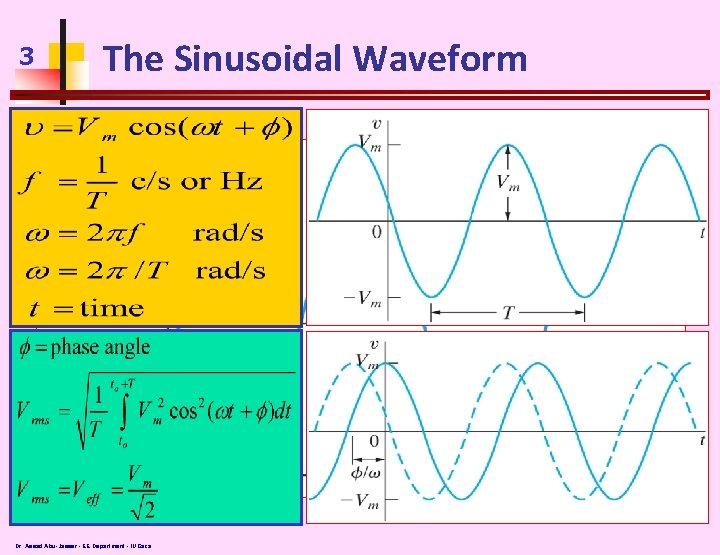3 The Sinusoidal Waveform Dr. Assad Abu-Jasser - EE Department - IUGaza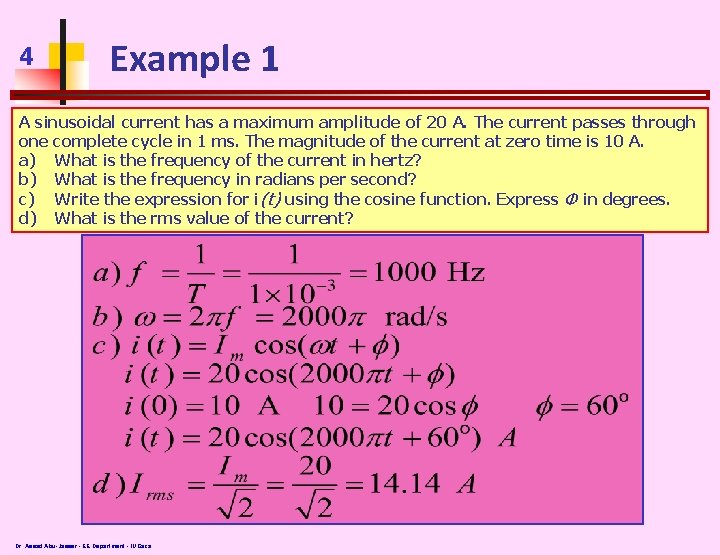4 Example 1 A sinusoidal current has a maximum amplitude of 20 A. The current passes through one complete cycle in 1 ms. The magnitude of the current at zero time is 10 A. a) What is the frequency of the current in hertz? b) What is the frequency in radians per second? c) Write the expression for i(t) using the cosine function. Express Φ in degrees. d) What is the rms value of the current? Dr. Assad Abu-Jasser - EE Department - IUGaza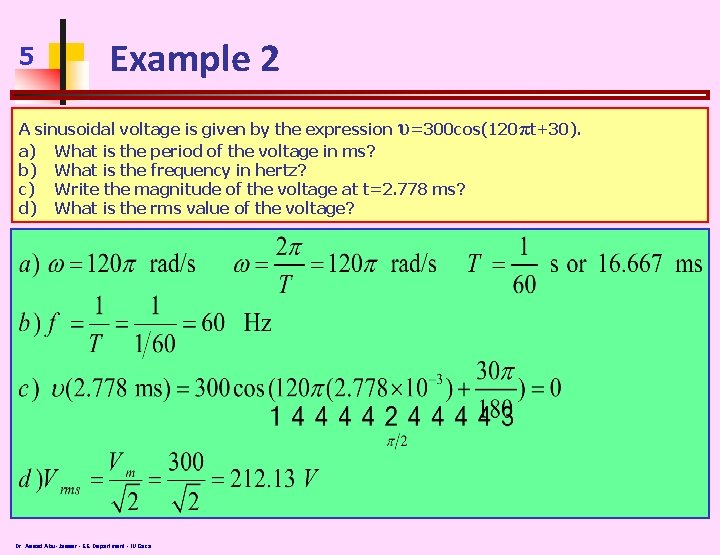5 Example 2 A sinusoidal voltage is given by the expression υ=300 cos(120πt+30). a) What is the period of the voltage in ms? b) What is the frequency in hertz? c) Write the magnitude of the voltage at t=2. 778 ms? d) What is the rms value of the voltage? Dr. Assad Abu-Jasser - EE Department - IUGaza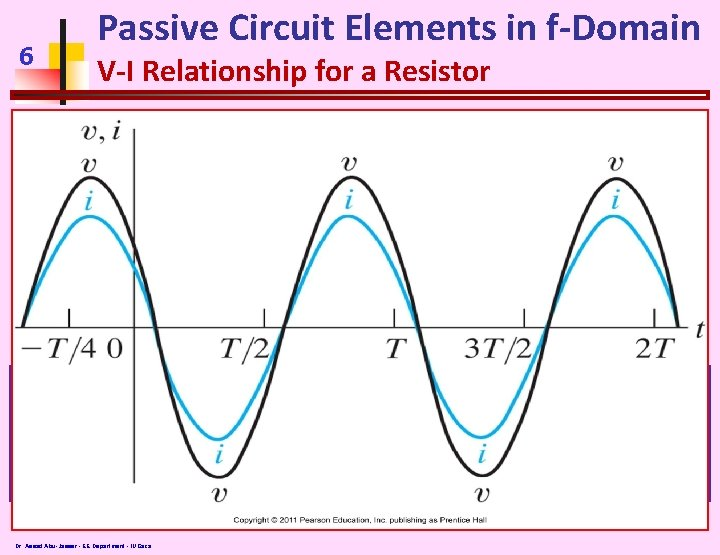6 Passive Circuit Elements in f-Domain V-I Relationship for a Resistor Dr. Assad Abu-Jasser - EE Department - IUGaza7 Passive Circuit Elements in f-Domain V-I Relationship for an Inductor Dr. Assad Abu-Jasser - EE Department - IUGaza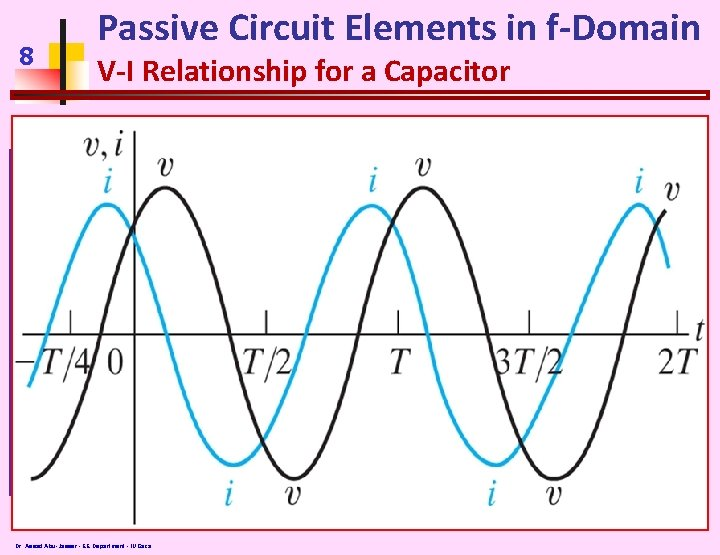8 Passive Circuit Elements in f-Domain V-I Relationship for a Capacitor Dr. Assad Abu-Jasser - EE Department - IUGaza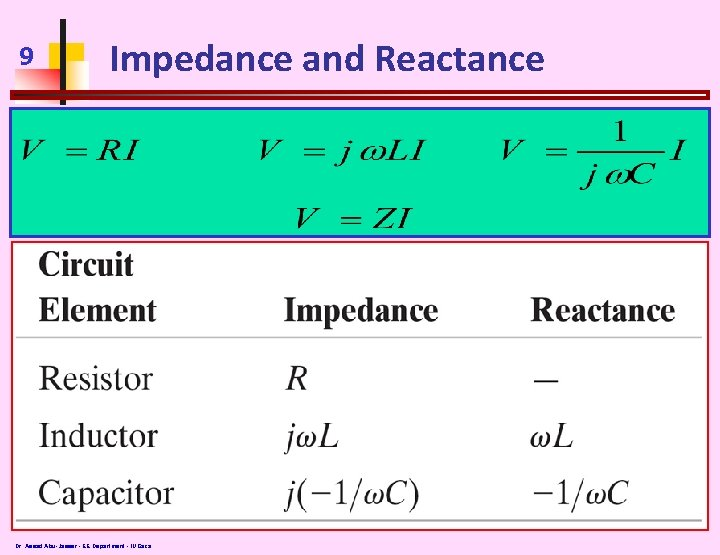9 Impedance and Reactance Dr. Assad Abu-Jasser - EE Department - IUGaza10 Example 3 A 90Ω resistor, a 32 m. H inductor, and a 5 μF capacitor are connected in series across the terminals of a sinusoidal voltage source. The steady-state expression for the source voltage υs is 750 cos(5000 t+30 o) V. a) Construct the frequency-domain equivalent circuit b) Calculate the steady-state current i by the phasor method Dr. Assad Abu-Jasser - EE Department - IUGaza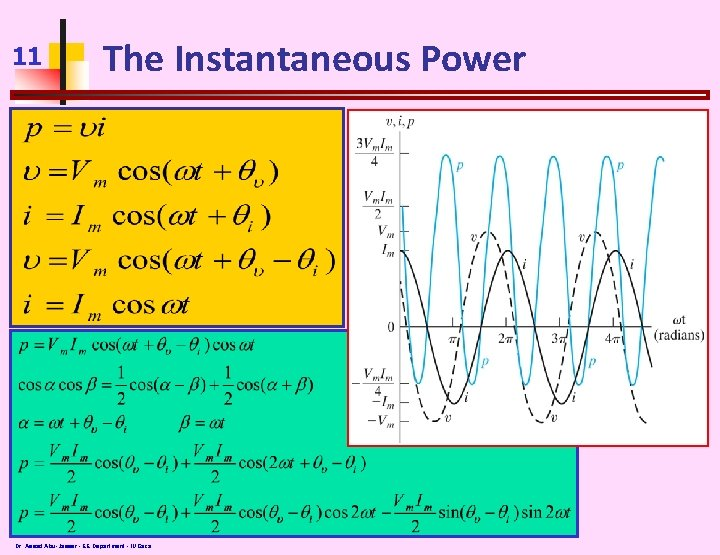11 The Instantaneous Power Dr. Assad Abu-Jasser - EE Department - IUGaza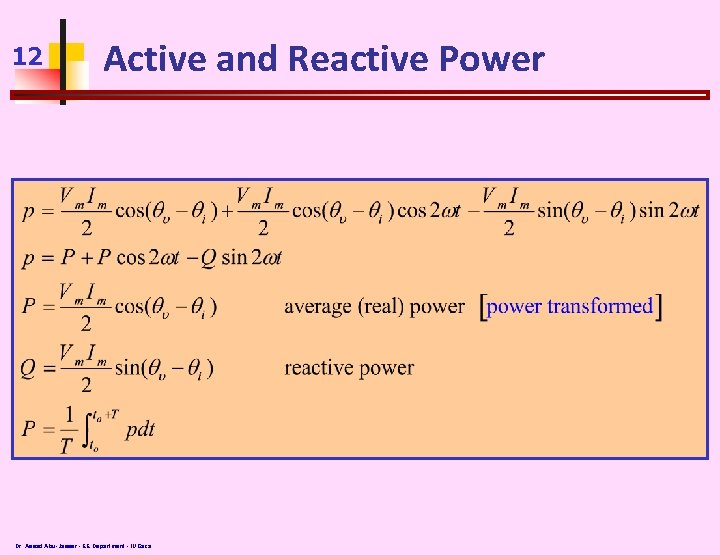12 Active and Reactive Power Dr. Assad Abu-Jasser - EE Department - IUGaza13 Power for Purely Resistive Circuit Dr. Assad Abu-Jasser - EE Department - IUGaza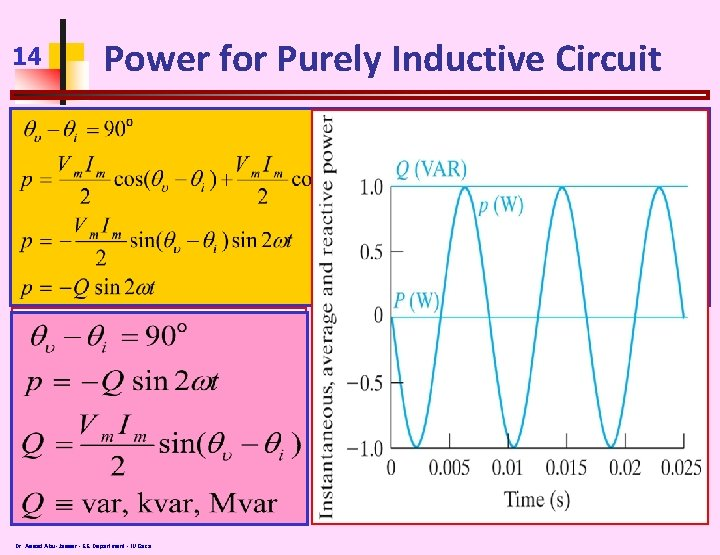14 Power for Purely Inductive Circuit Dr. Assad Abu-Jasser - EE Department - IUGaza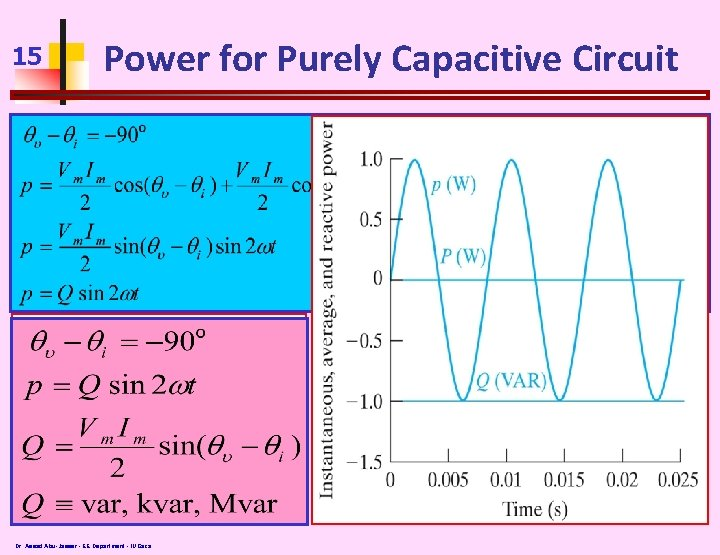15 Power for Purely Capacitive Circuit Dr. Assad Abu-Jasser - EE Department - IUGaza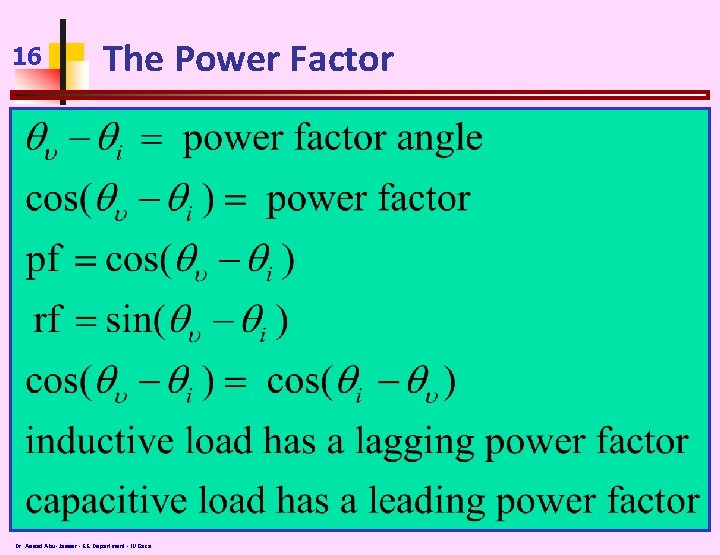16 The Power Factor Dr. Assad Abu-Jasser - EE Department - IUGaza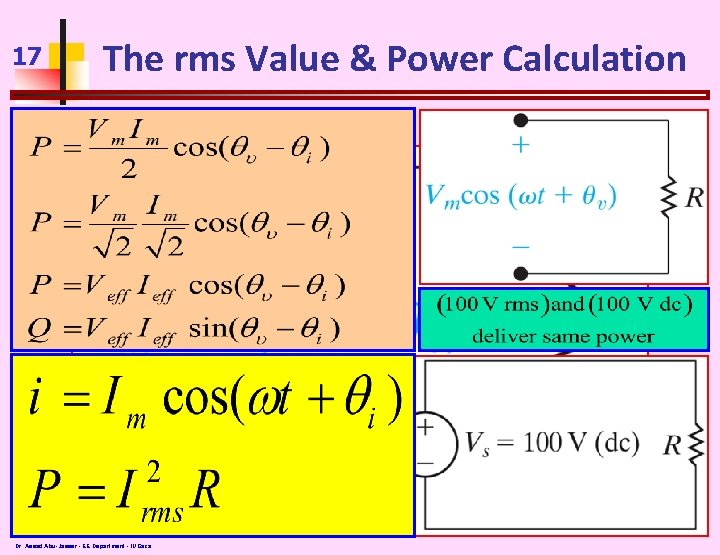17 The rms Value & Power Calculation Dr. Assad Abu-Jasser - EE Department - IUGaza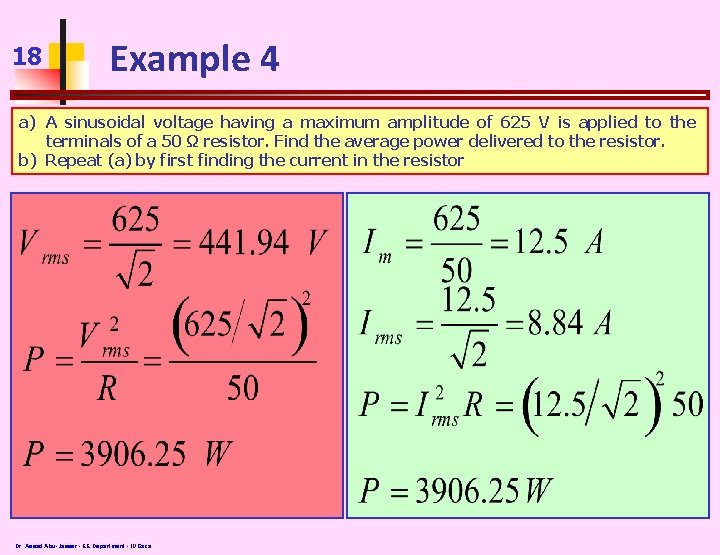18 Example 4 a) A sinusoidal voltage having a maximum amplitude of 625 V is applied to the terminals of a 50 Ω resistor. Find the average power delivered to the resistor. b) Repeat (a) by first finding the current in the resistor Dr. Assad Abu-Jasser - EE Department - IUGaza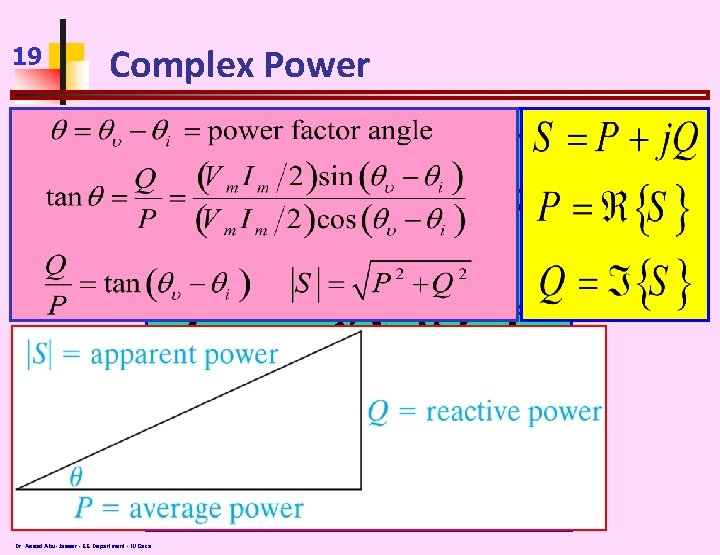19 Complex Power Dr. Assad Abu-Jasser - EE Department - IUGaza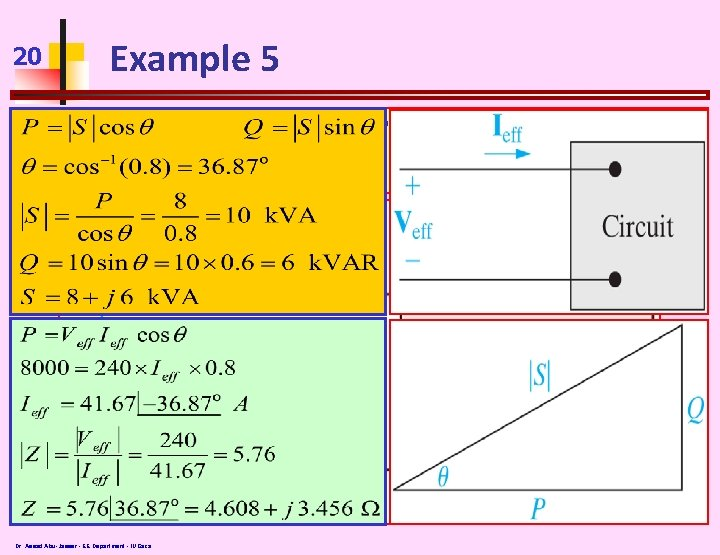20 Example 5 An electric load operates at 240 V rms. The load absorbs an average power of 8 k. W at 0. 8 lagging power factor. a) Calculate the complex power of the load b) Calculate the impedance of the load Dr. Assad Abu-Jasser - EE Department - IUGaza21 Balanced Three-Phase Voltages Dr. Assad Abu-Jasser - EE Department - IUGaza22 Three-Phase Voltage Sources Source Y Y ∆ ∆ Dr. Assad Abu-Jasser - EE Department - IUGaza Load Y ∆23 Analysis of the Wye-Wye Circuit Va’n = Vb’n= Vc’n Zga=Zgb=Zgc Z 1 a=Z 1 b=Z 1 c ZA=ZB=ZC Dr. Assad Abu-Jasser - EE Department - IUGaza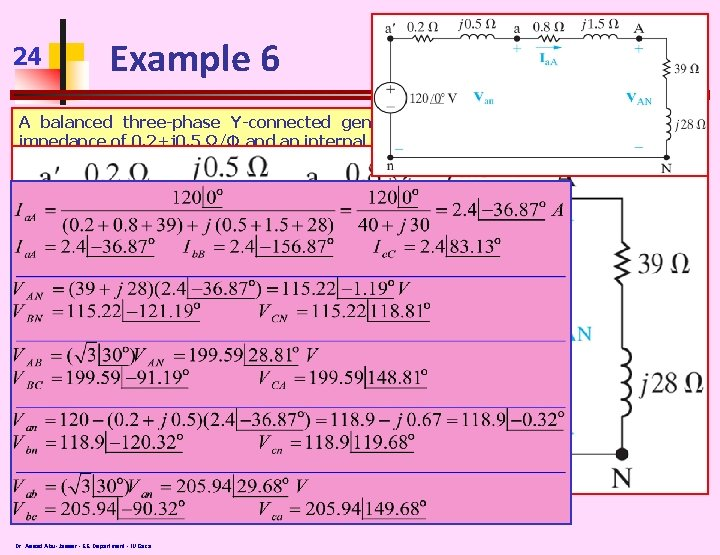24 Example 6 A balanced three-phase Y-connected generator with positive sequence has an impedance of 0. 2+j 0. 5 Ω/Φ and an internal voltage of 120 V/Φ. The generator feeds a balanced three-phase Y-connected load having an impedance of 39+j 28 Ω/Φ. The impedance of the line connecting the generator to the load is 0. 8+j 1. 5 Ω/Φ. The aphase internal voltage of the generator is specified as the reference phasor. a) Construct the a-phase equivalent circuit of the system b) Calculate three line currents Ia. A, Ib. B, and Ic. C c) Calculate three phase voltages at the load, VAN, VBN, and VCN d) Calculate the line voltages , VAB, VBC, and VCA at the terminals of the load e) Calculate the phase voltages at the generator terminals, V an, Vbn, and Vcn f) Calculate the line voltages at the generator terminals, V ab, Vbc, and Vca Dr. Assad Abu-Jasser - EE Department - IUGaza25 End Dr. Assad Abu-Jasser - EE Department - IUGaza